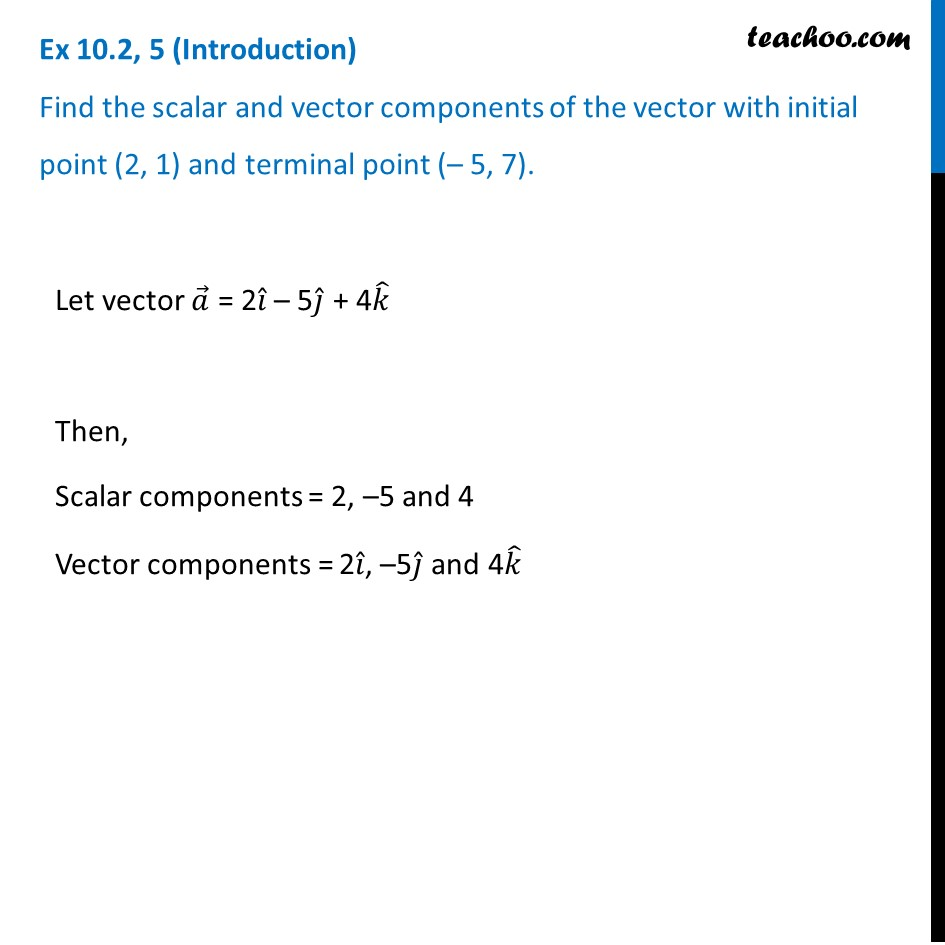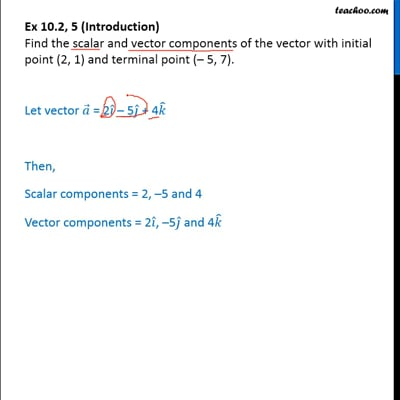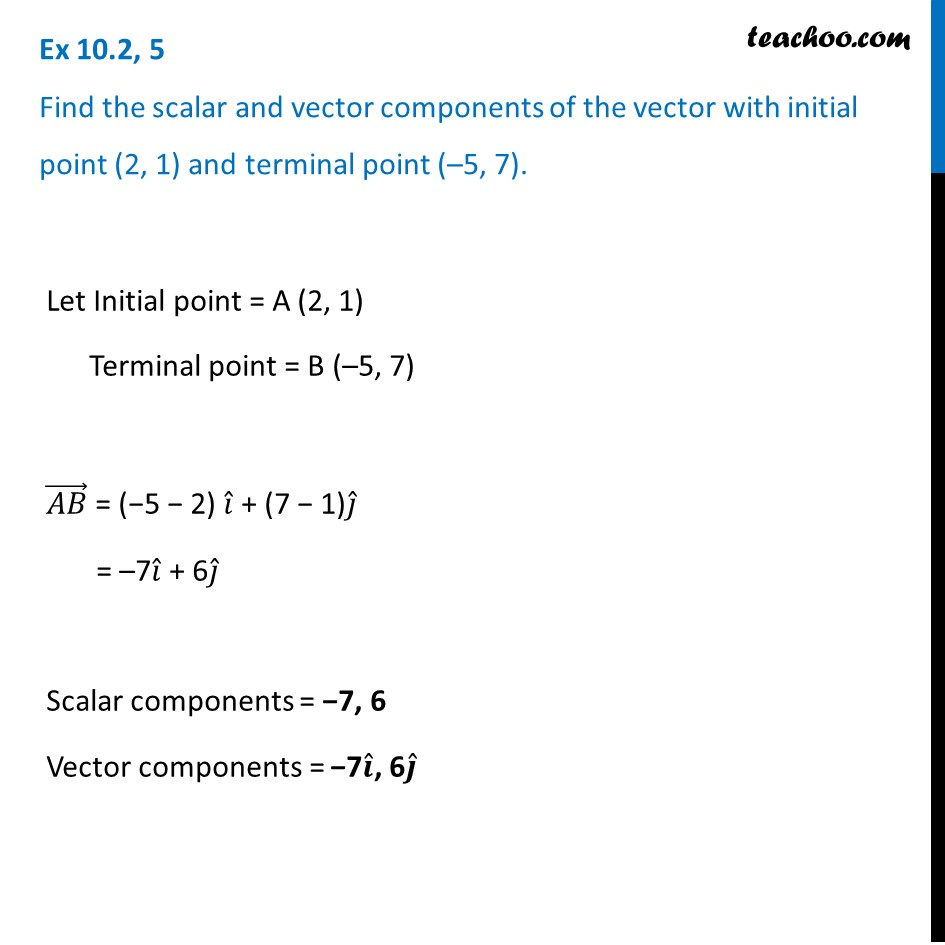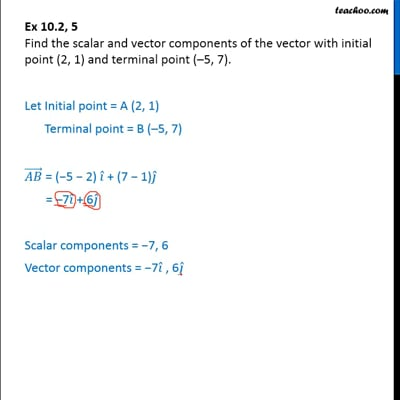Ex 10.2

Chapter 10 Class 12 Vector Algebra
Serial order wiseThis video is only available for Teachoo black usersThis video is only available for Teachoo black users

Solve all your doubts with Teachoo Black (new monthly pack available now!)

### Transcript

Ex 10.2, 5 (Introduction) Find the scalar and vector components of the vector with initial point (2, 1) and terminal point (– 5, 7). Let vector 𝑎 ⃗ = 2𝑖 ̂ – 5𝑗 ̂ + 4𝑘 ̂ Then, Scalar components = 2, –5 and 4 Vector components = 2𝑖 ̂, –5𝑗 ̂ and 4𝑘 ̂ Ex 10.2, 5 Find the scalar and vector components of the vector with initial point (2, 1) and terminal point (–5, 7). Let Initial point = A (2, 1) Terminal point = B (–5, 7) (𝐴𝐵) ⃗ = (−5 − 2) 𝑖 ̂ + (7 − 1)𝑗 ̂ = –7𝑖 ̂ + 6𝑗 ̂ Scalar components = −7, 6 Vector components = −7𝒊 ̂, 6𝒋 ̂